• 검색 결과가 없습니다.

# Eunyeong Yang

N/A
N/A
Protected

Share "Eunyeong Yang"

Copied!
38
0
0
더 보기 ( 페이지)

전체 글

One of the ferroelectric-based NVMs is a ferroelectric tunnel junction (FTJ), which consists of ferroelectric material sandwiched between conductive materials on both sides, such as metal or highly doped semiconductors. The FTJ works as a tunable resistance device by modulating the tunnel potential barrier height by reversing its inherent polarization. To investigate the performance of the FTJ theoretically, we first calculate the real and complex band structures of HZO and CIPS using density functional theory (DFT) via Atomistic ToolKit (ATK) simulator.

Then, for efficiency in simulating the device, a two-band 𝑘‧𝑝 Hamiltonian is established by fitting the first band to the complex band structures from DFT. The MFM-FTJ and MFIM-FTJ potential profiles are a self-consistent calculation using Thomas-Fermi screening, P-V fitting model and Poisson's equation. We then build the transfer matrix to calculate the transport and calculate the transfer in the FTJ for a given bias.

We study the dependence of TER as a function of ferroelectric thickness tFE and polarization Ps to investigate the conditions for obtaining highly enhanced TER with excellent ON/OFF detection. Initial band offsets of (a) CIPS and (b) HZO MFM FTJ. a) Procedure and (b) equations for obtaining the self-consistent potential profile in the MFM FTJ. Initial band offsets of (a) CIPS and (b) HZO MFIM FTJ. a) Procedure and (b) equations for obtaining the self-consistent potential profile in the MFIM FTJ.

## Introduction

### Ferroelectric Materials

According to the Landau-Devonshire phase transition model , ferroelectric materials show characteristic nonlinear hysteresis of the P polarization in accordance with the applied field. Depending on the applied field in ferroelectric materials, the localized P group gradually aligns with the direction of the external field. When the applied electric field reaches above the compressive field (Ec), the total polarization direction eventually changes.

With this electrical switching and residual polarization behavior, this ferroelectricity has been explored for the implementation of non-destructive and permanent memory devices. Materials such as BaTiO3 [3-6] and PbZrTiO3 [7, 8] have been extensively investigated to exhibit a ferroelectric phase even below a few unit cells. The MTP family consists of a metal cation and a [P2S6]4- framework, and is also in a multilayer structure connected by weak van der Waals forces, which enables the realization of a finite 2D ferroelectric system.

We focus on ferroelectric CuInP2S6 (CIPS) in the MTP group, which exhibits ferroelectricity in a certain non-centrosymmetric monoclinic phase. These nanometer-thick 2D ferroelectric CIPS have the advantage of being useful for flexible, highly integrated, low-power devices.

Ferroelectric Tunnel Junctions

## Investigation of Ferroelectric Material Properties by Density Functional Theory

• Crystal Structure of Bulk CuInP 2 S 6
• Crystal Structure of Bulk HfZrO 2
• Band Structure of 2D CuInP 2 S 6
• Band Structure of HfZrO 2
• Complex Band Structures of 2D CuInP 2 S 6 and HfZrO 2

The pseudopotential is carefully chosen to obtain a value of the band gap relative to the experimentally measured one. The band structure is calculated using the PseudoDojo and SG15 pseudopotentials with a lattice cutoff energy of 105 and 200 Hartree, respectively. In order to observe the band structure at a particular ferroelectric phase, we confirm that the CIPS bulk crystal structure is fixed with monoclinic Cc symmetry and fully relaxed until the maximum force of each atom is less than 0.05 eV/Å.

Then, with the geometrically optimized structure, the actual band structure is calculated together with the high symmetry points in the 1st Brillouin zone (BZ), as shown in Fig. showed. Since the previously reported band structure of CIPS has a direct band gap at Γ with Eg. =2.9 eV , we adopt the PseudoDojo method for further calculation. To investigate the band structure at a certain ferroelectric phase, the bulk crystal structure of HZO in orthorhombic phase is fixed with Pca21.

Using a geometrically optimized structure, the band structure of ferroelectric HZO is calculated together with high symmetry points within the 1st BZ. The band structures with PseudoDojo and SG15 have similar band gap sizes of Eg = 3.9 eV, and the VBM is located at the Γ point. Since FTJ devices mainly generate current in which electrons tunnel vertically through the ferroelectric layer, it is important to accurately characterize the tunneling properties by investigating the complex band structure in the tunneling direction.

The complex band structure shows the imaginary values ​​of kz with respect to the energy E obtained by solving the eigenvalue problem. The complex band structures of CIPS and HZO are calculated via the z-direction kz in reciprocal coordinates as shown in the figure. As shown by the previous Bloch function, the evanescent mode with the smallest kz would decay less.

Therefore, only the first band with the smallest kz is used for transmission calculation. Even the innermost band edge connects the CBM and VBM in the actual band structures with a band gap of Eg=1.7 eV and Eg=3.9 eV for CIPS and HZO, respectively.

## Theoretical Calculation of FTJ

• Simulation Workflow of Modeling FTJ
• Fitting Complex Band Structure DFT and 𝑘 ∙ 𝑝 Hamiltonian
• Self-consistent Potential Calculation
• Quantum Transport Calculation

Since we consider the tunneling current in the z direction, we investigate the complex band structures composed of the modes evident in the bandgap through the z direction in reciprocal coordinates. Among the complex bands, the innermost band with the smallest kz connecting the CBM and VBM in the real band structures dominates the tunneling current because the evanescent mode with the smallest k decays less. Therefore, focusing only on the band with the smallest kz is sufficient to consider the transport calculation.

9(a) and (b), the fitting parameters are carefully chosen as v=4.0×105 and v=7.8×105 for CIPS and HZO to reproduce the first band in the complex band structures from DFT. TiN and Pt metals are applied to each bottom and top electrode with metal work function 𝛷m1=4.7 eV and 𝛷m2=5.3 eV, respectively . The robust computation starts with the arbitrary sum of the screening fee Q and the applied bias 𝑉a.

Until those values ​​become equivalent, the margin ∆Q is augmented by the feedback loop and converged by the self-consistent calculation. Following the above workflow, we can finally obtain the self-consistent potential profiles of MFM FTJ, finalized as Fig. Therefore, the initial band deposits of MFIM FTJ for CIPS and HZO are calculated analytically as shown in Fig.

The term of the potential drop in the oxide layer, Vox is calculated by Poisson's equation in eq.1. We perform ballistic quantum transport calculations for MFM and MFIM FTJs using the k∙ p Hamiltonian and the self-consistent potential profiles. We solve the numerical 2D Schrödinger equation by cutting the device with the spacing of a =1Å in the transport z direction.

To deal with tunneling through an abrupt heterostructure metal-ferroelectric interface, we supplement the interface matrices using a position-dependent effective mass Hamiltonian . Then, the total flux is calculated by integrating the transfer probability with the weight of the Fermi function in the Landauer–Büttiker formalism [ 24 ].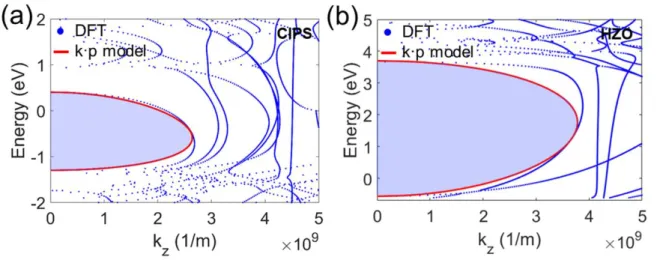Fig. 9. Complex band fitting of (a) CIPS, and (b) HZO between DFT and k∙p model.

## Tunneling Current and Device Performance of FTJ

• Tunneling Current of MFM-FTJ
• Tunneling Electroresistance of MFM-FTJ
• Tunneling Current of MFIM-FTJ
• Tunneling Electroresistance of MFIM-FTJ
• WKB Approximation

Tunnel electrical resistance (TER) is the difference between ON and OFF states determined by the following definition, TER = IONI-IOFF. 18(a) and (b) are 3D plots of the thickness and polarization change of TER calculated in CIPS FTJ and HZO FTJ. TER of CIPS FTJ increases exponentially and shows TER=111.11 and reaches 2 orders higher than TER=1.24 of HZO FTJ at 4.5 nm thick.

An advantage of the MFIM FTJ is that the thin oxide layer between the ferroelectric and the metal enhances asymmetric scanning effects. All parameters of the ferroelectric layers, Ps and Vc of the ferroelectric layers, are identical with 8 and 20 μC/cm2, 0.14 and 0.1 MV/cm for CIPS and HZO, respectively. Below the dominant forward tunnel current range, a small readout voltage Va = ±0.5 V is applied to the MFIM FTJs.

To calculate transport through an additional thin oxide layer, the effective mass of the SiO2 layer in the z-direction refers to the experimentally measured values ​​moxc=0.427m0 and moxv=0.573m0 . Therefore, the depolarization field is effectively increased, and the modulation of the barrier in the ON and OFF states is improved in the MFIM FTJ than in the MFM FTJ. The additional thin oxide layer results in approximately two orders of magnitude lower tunnel current level than in MFM FTJs.

20(a) and (b), the TER values ​​of MFIM FTJ are shown along with the change in thickness and polarization of the ferroelectric layer. In particular, the improved ON and OFF barrier modulation of the ferroelectric layer results in an overall increase in the TER despite the lower tunnel current level. The reason why the TER is higher with CIPS FTJ can be explained by the relatively low potential barrier between metal and CIPS.

Since the electron affinity of CIPS is greater than HZO, the average barrier height 𝑉0 is lower in CIPS FTJ than in HZO FTJ. Therefore, the TER of CIPS FTJ can grow higher and faster with increasing thickness and polarization, as shown in Fig.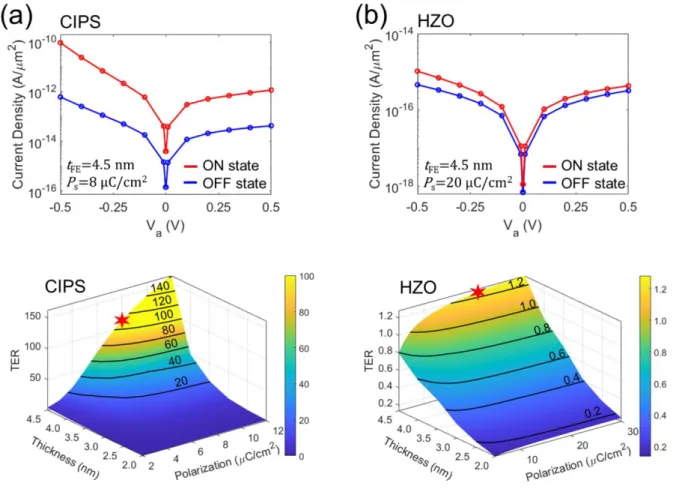Fig. 18. TER of (a) CIPS, and (b) HZO MFM FTJ with the variation of thickness and polarization

## Conclusion

Cao, “Atomic-scale deposition to extend Moore's law and beyond,” International Journal of Extreme Manufacturing , vol. Gruverman et al., "Electroresistivity effect of tunneling in ferroelectric tunnel junctions at the nanometer," Nano letters, vol. Ming, “Electric resistivity enhanced by ferroelectric field effect in metal/ferroelectric/semiconductor tunnel junctions,” Nature materials , vol.

Xi et al., “Giant tunnel electroresistance in metal/ferroelectric/semiconductor tunnel junctions by Schottky barrier engineering,” Nature Communications , vol. Ueda, "Pyroelectric properties and application in infrared sensors of PbTiO3, PbLaTiO3 and PbZrTiO3 ferroelectric thin films", Ferroelectrics, vol. Pantel et al., "Tunnel electrical resistance in junctions with ultrathin ferroelectric Pb(Zr0.2Ti0.8)O3 barriers", Applied Physics Letters, vol.

Maksymovych, “Metal thio- and selenophosphates as multifunctional van der Waals multilayer materials,” Advanced Materials , vol. Wu et al., “High tunneling resistivity in a ferroelectric van der Waals heterojunction via massive barrier height modulation,” Nature Electronics , vol. Sun, “Ultrathin two-dimensional vertical ferroelectric tunnel junction based on CuInP2S6 monolayer,” Nanoscale , vol.

Studenyak et al., "Disturbing effect on optical absorption processes in CuInP2S6 layered ferrielectrics," physica status solidi (b), vol. Ambriz-Vargas et al., "A complementary metal-oxide-semiconductor process-compatible ferroelectric tunnel junction," ACS Applied Materials and interfaces, vol Yokoyama, "Numerical analysis of ballistic electron transport in magnetic fields using a quantum dot contact and a quantum wire."

Kobayashi, "Study of ferroelectric-HfO2 tunnel junction memory scalability based on non-equilibrium Green's function method". Chanana, “BOEMDET band gaps and effective mass determination technique applied to MIS devices on silicon to obtain unknown band gap of insulators,” IOSR Journal of Applied Physics, vol.

수치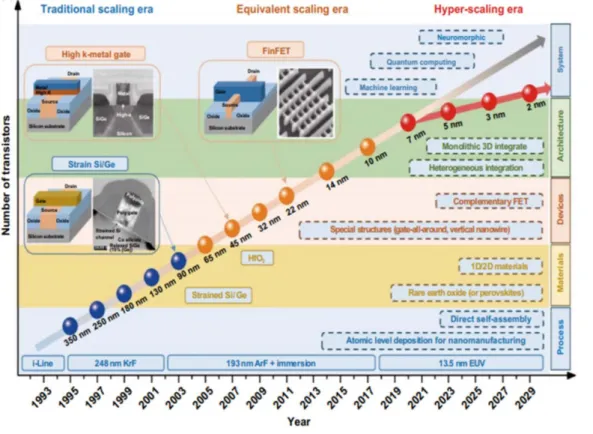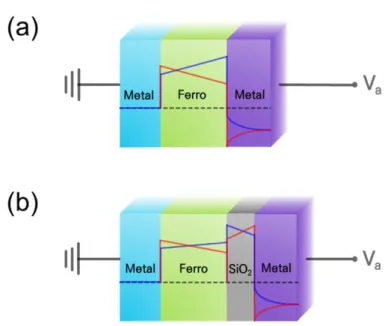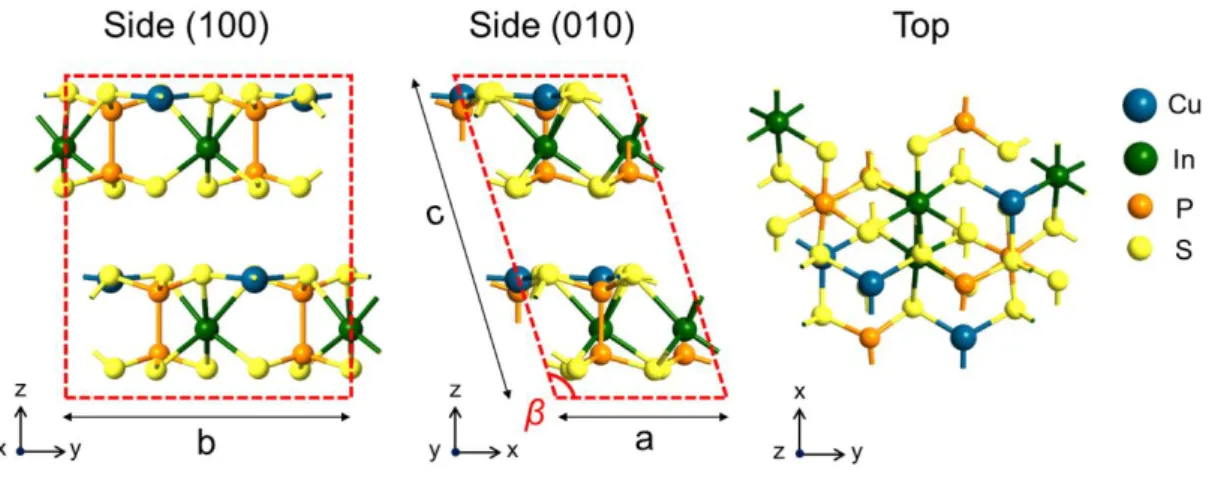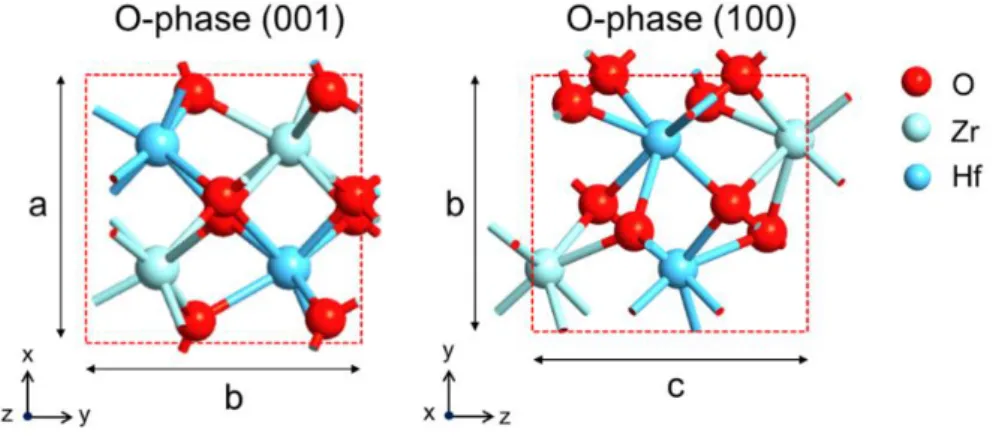+7

참조

Outline

관련 문서

Against the background of the uncertain international economic environment, the year 2012 has seen numerous shocks in the Nigerian economy some of which

If plasmonic waveguides are intended to propagate light in subwavelength modes, MIM geometries with higher confinement factors and shorter spatial extents are much better

• Guaranteed payment of medical expenses for high-cost medical treatment. 5 미소심는치과 Dental Disease •according to Medical insurance (paid-medical

1) OLED의 Full-Color 방법.. Transparent anode electrode patterning.

cd/W Luminous flux per sr per input electrical unit power Luminous intensity efficiency. lm/W Luminous flux per input

In this paper, we have analyzed the effect of digital power/ground noise coupling on the performance of active balun of RF circuit with aspects of both on-chip substrate

We see that for the three countries contributing the most to developing country remittance growth over this period—India, China, and Nigeria—that growth in migrant stocks and

우선, 자동차산업의 약 30여개 유관기관이 상호 협력하여 자동차관리정보 시스템을 구축하였다는 점은 양식어업에서도 이러한 유관기관 간의 상호 협 력을 통해 양식정보를

Four case studies were selected (i) cyclic volatile methyl siloxanes (cVMSs) in the Inner Oslofjord, (ii) cVMSs in the Norwegian Arctic, (iii) short- chain

언어 선택하기

웹사이트는 선택한 언어로 번역됩니다.

기타 언어